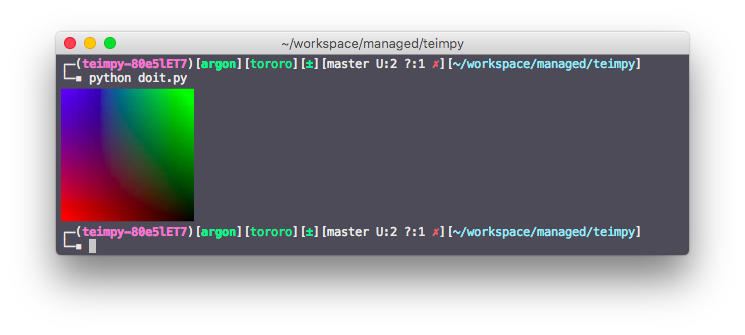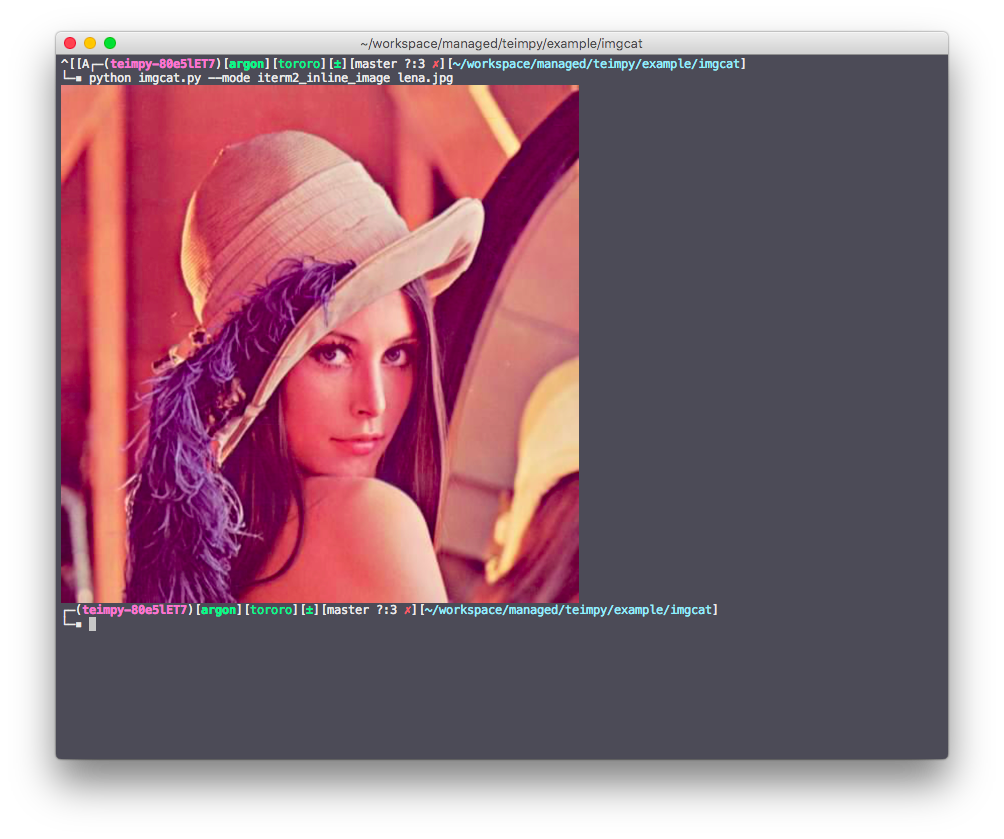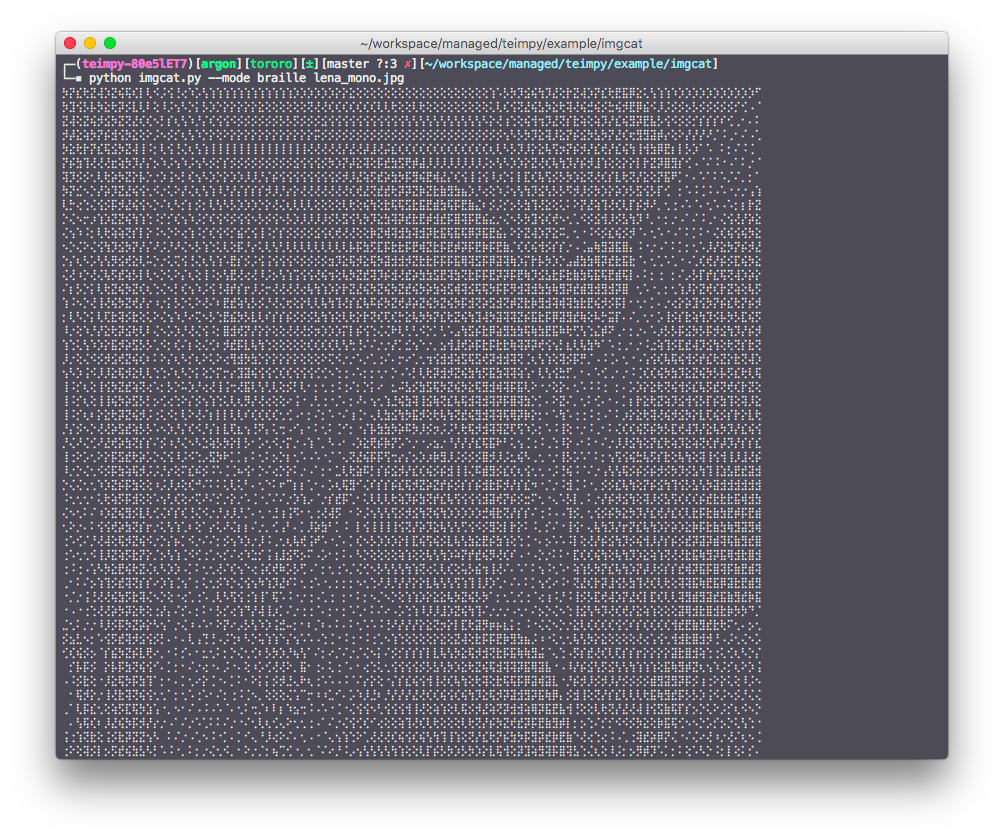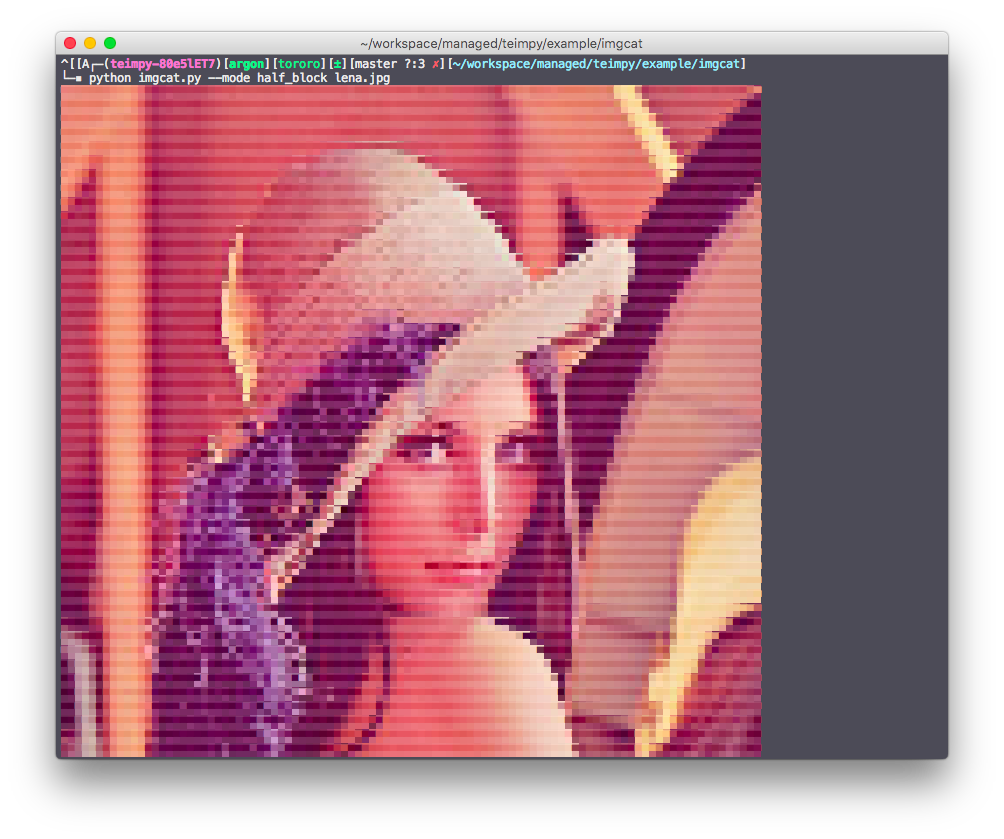Python libray for displaying images on terminal

# teimpy

Python library for displaying image on terminal.

## Installation

\$ pip


## Example

import numpy as np
from teimpy import get_drawer, Mode

R = np.array([1.0, 0, 0])
G = np.array([0, 1.0, 0])
B = np.array([0, 0, 1.0])

def _get_color(x, y):
tmp = (y * (1.0 - x) * R + (1.0 -y ) * x * G + (1.0 - y) * (1.0 - x) * B)
return (255 * tmp).astype(np.uint8)

tics = np.linspace(0, 1, 128)
xs, ys = np.meshgrid(tics, tics)
buffer = np.vectorize(_get_color, signature='(),()->(3)')(xs, ys)
print(get_drawer(Mode.ITERM2_INLINE_IMAGE).draw(buffer))## Feature

• Resize images to fit terminal size.
• Drawing with iterm2 inline image.• Drawing with Braille fonts.• Drawing with half block fonts.## Project details

This version0.1.10.1.00.0.30.0.20.0.1

Uploaded source